Open in App
Not now

# Bar Chart in Tableau

• Last Updated : 01 Oct, 2020

In this article we will learn how to draw bar chart in tableau worksheet to do further operations. For this first look into two terms :

• Tableau : Tableau is a very powerful data visualization tool that can be used by data analysts, scientists, statisticians, etc. to visualize the data and get a clear opinion based on the data analysis. Tableau is very famous as it can take in data and produce the required data visualization output in a very short time.
• Bar Chart : It is a graph that displays categorical data with rectangular bars with heights that are equivalent to the amounts they depict. The bars can be displayed vertically or horizontally. A vertical bar map is often referred to as the column map.

Below are the steps to illustrate data in the form of a Bar chart in Tableau:

1) Open Tableau tool and connect a dataset into it.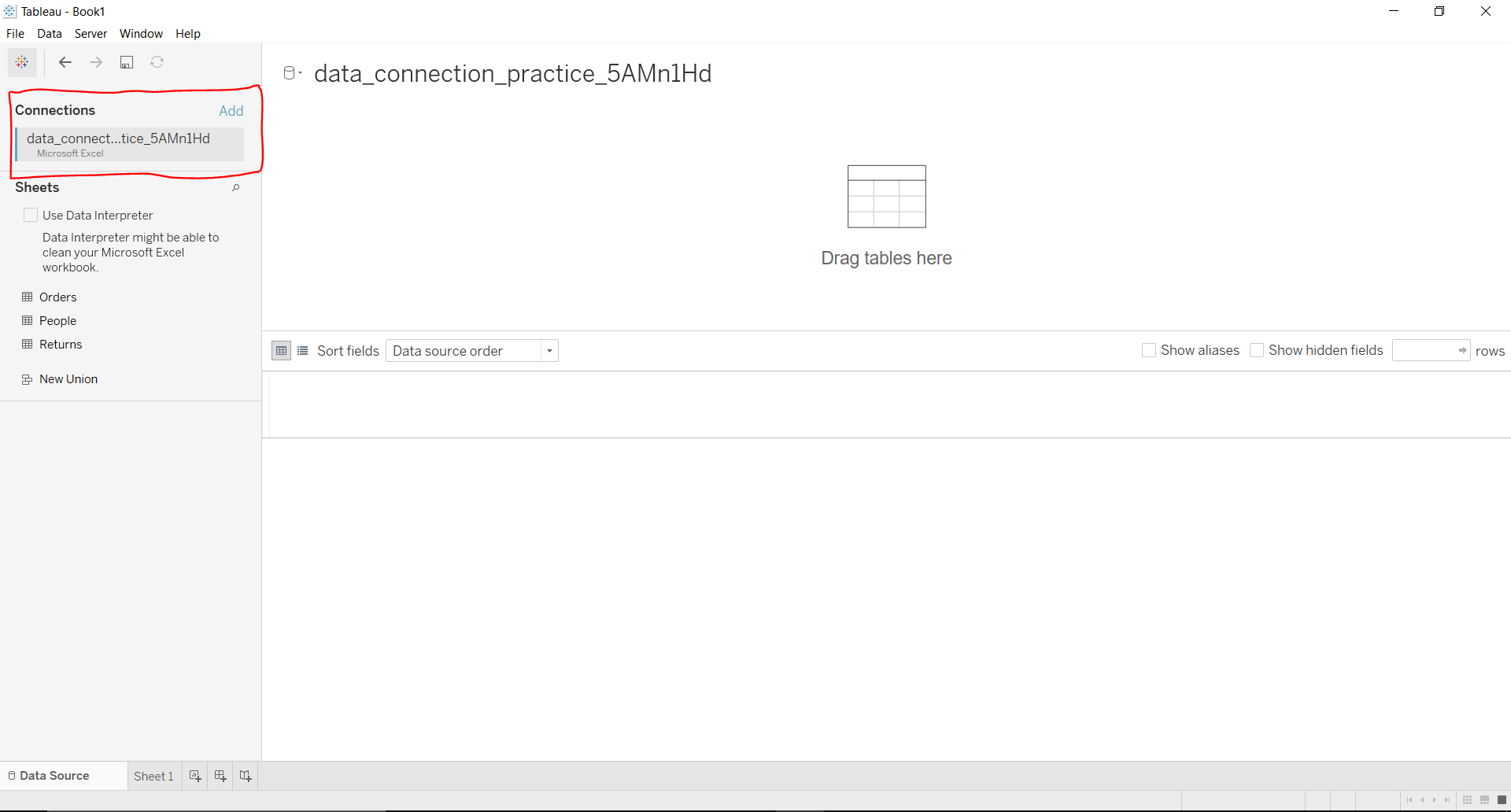2) Drag and drop the one sheet of connected dataset.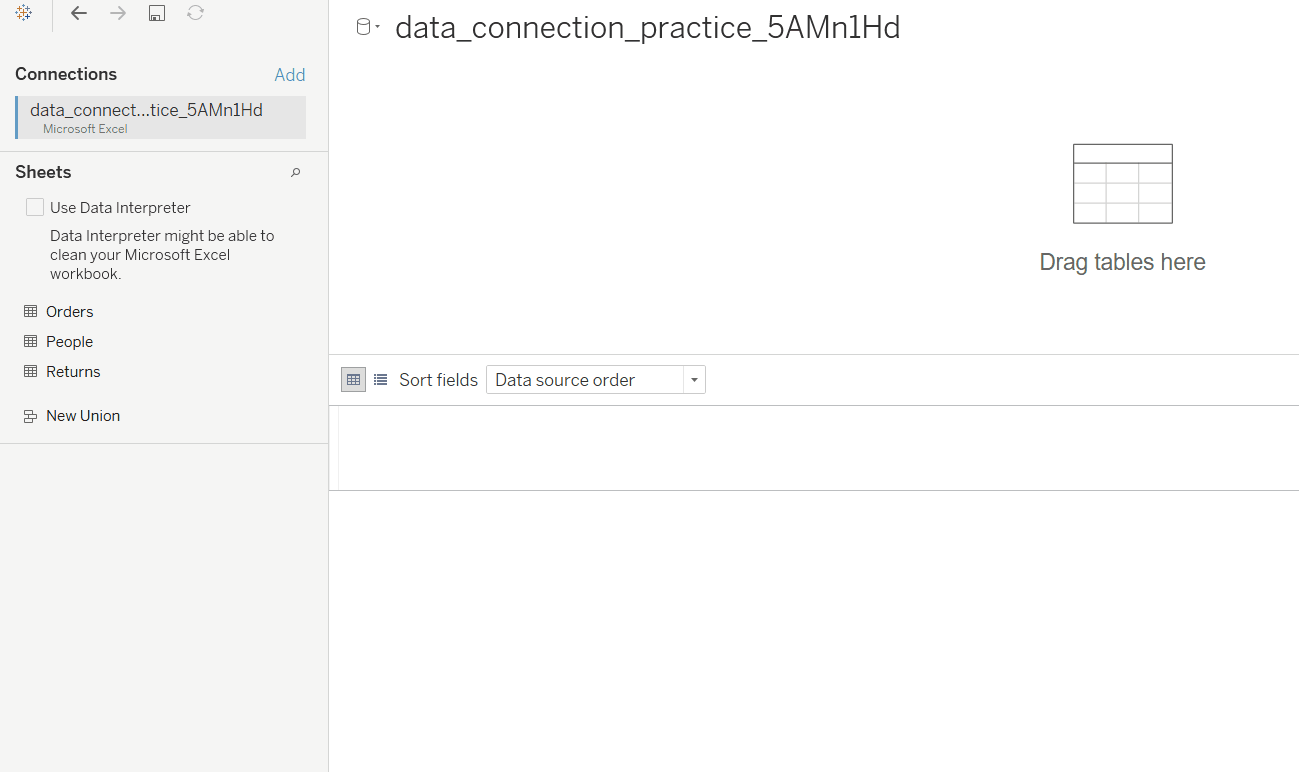3) Click on sheet1 to open the tableau worksheet.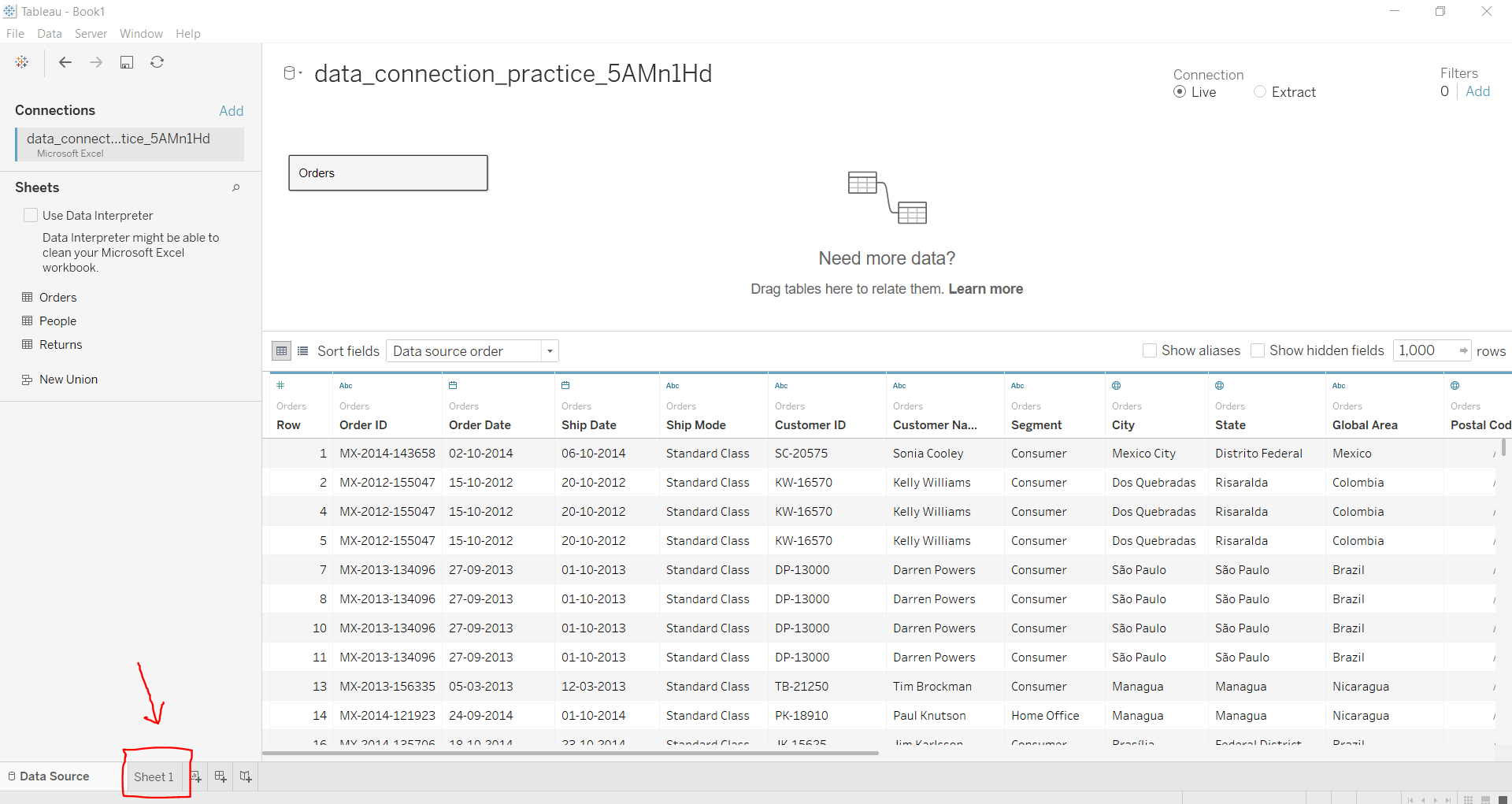4) On clicking Sheet1 you will get whole dataset attributes on left side and a worksheet for work.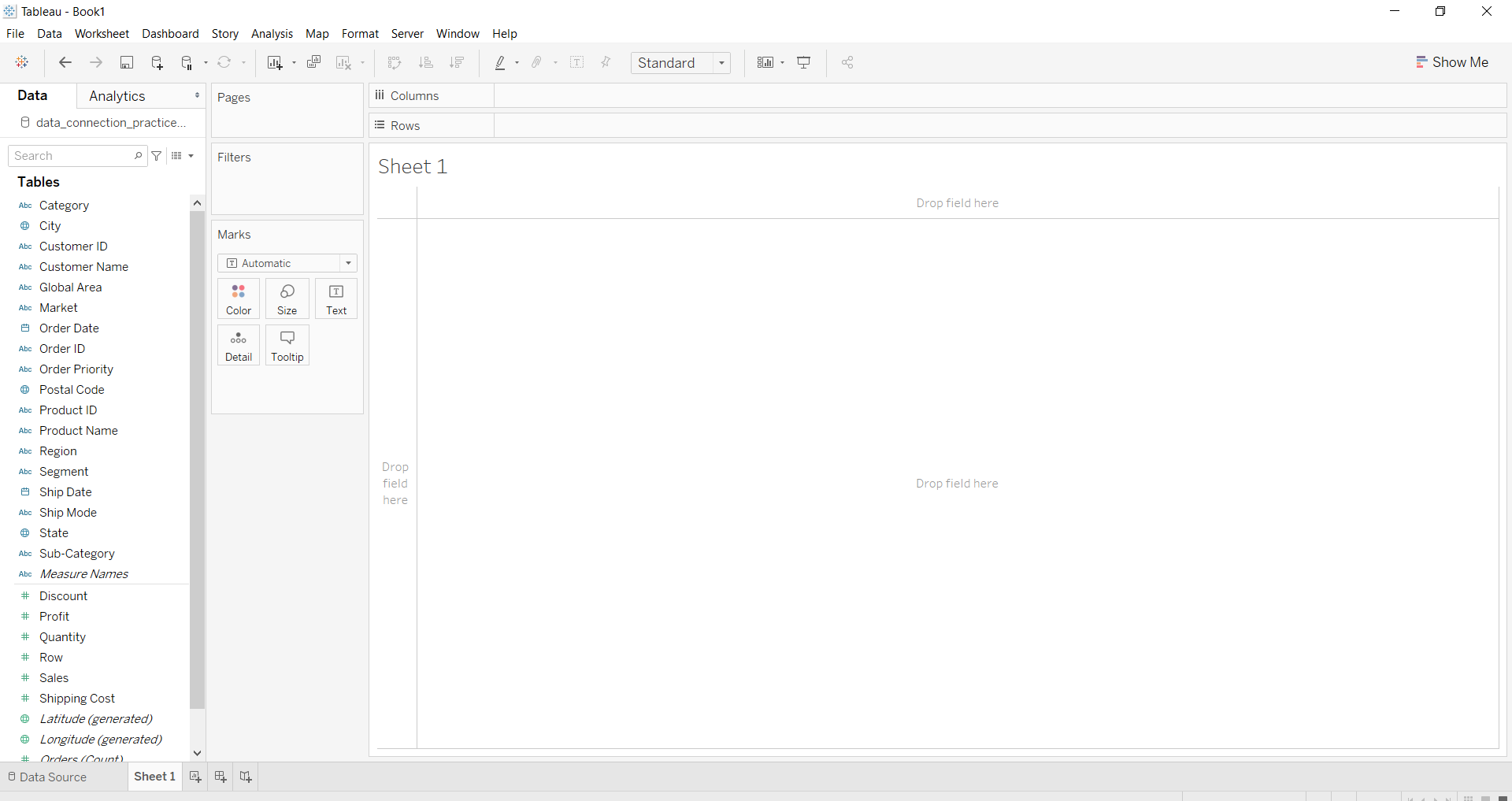5) To draw a bar chart you have to select minimum two attributes( one in row and one in column) by drag and drop then select the chart option as bar.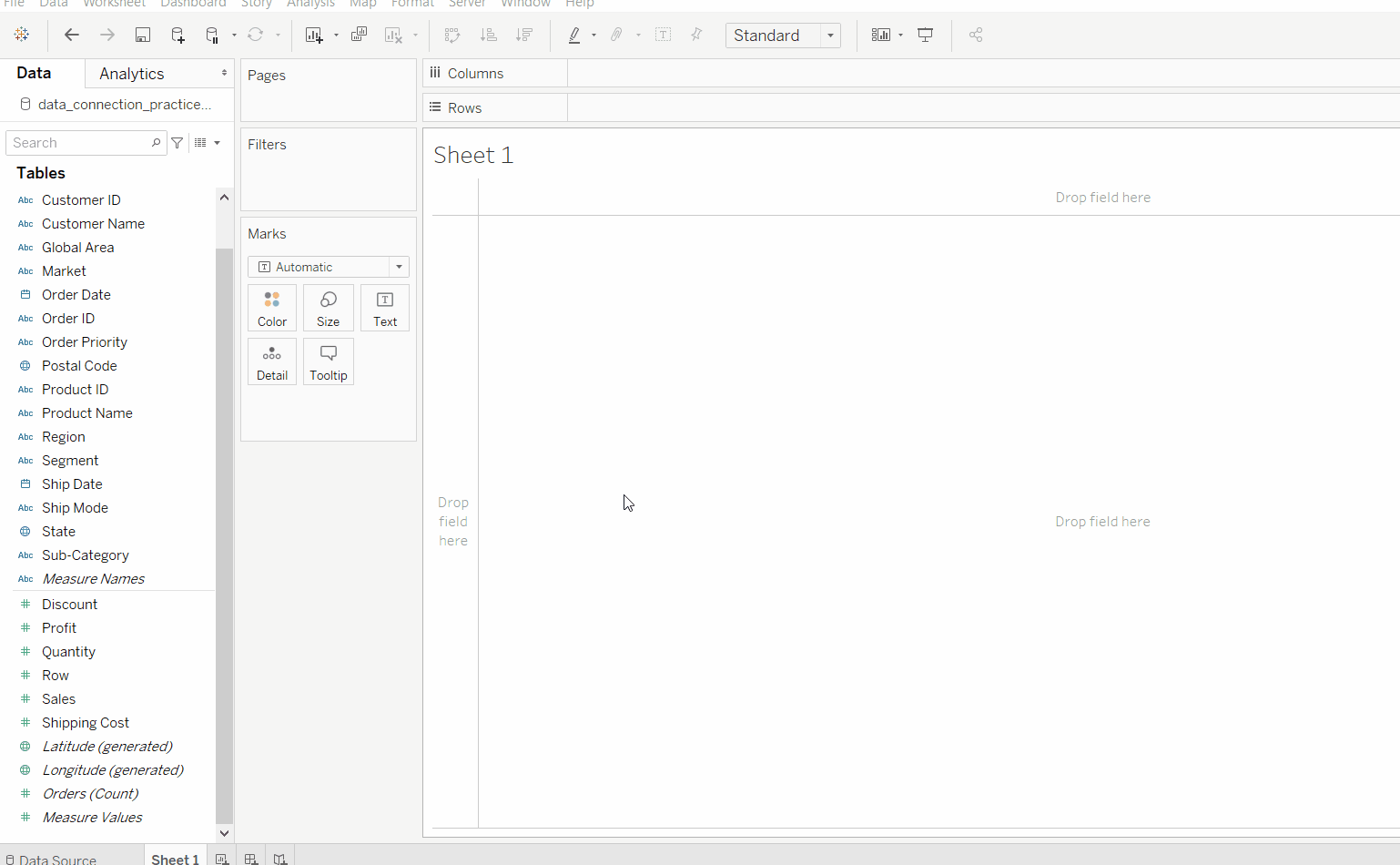### Below are some examples of the above approach:

Example 1:

This example is also drawn similar to the above steps with some extra marks and which is explained in steps given below:

• Select the Date (dimension must be like year, month, etc ) and Sales with profit fields as column and rows respectively.
• Select the chart type bar chart.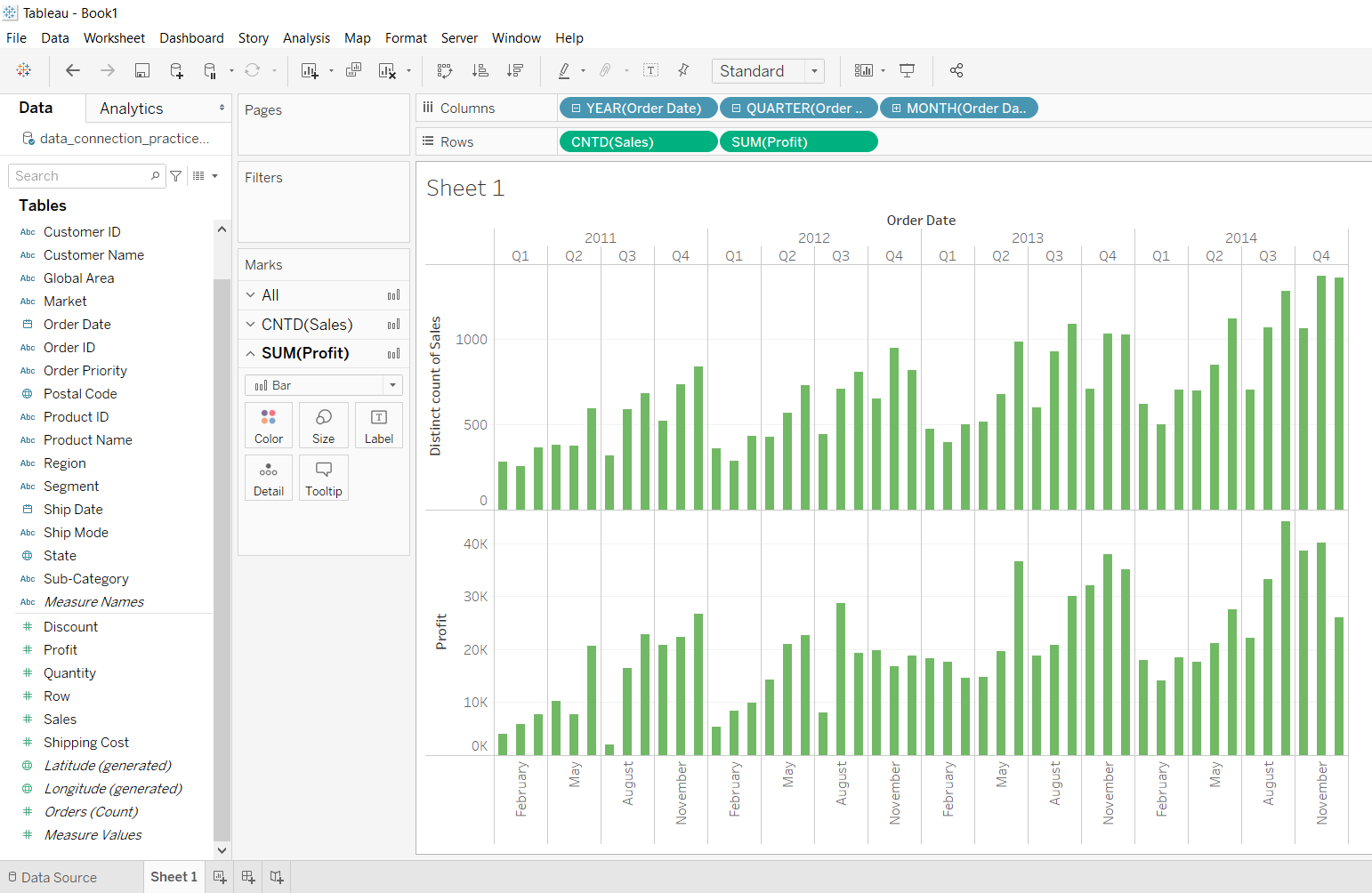Example 3:

This example is also drawn similar to above example 2 with some extra marks and which is explained in steps given below:

• Select the Region and Sales with profit fields as row and columns respectively.
• Select the chart type bar chart.
• Apply marks by color of discount.
• Apply filter by sum of quantity.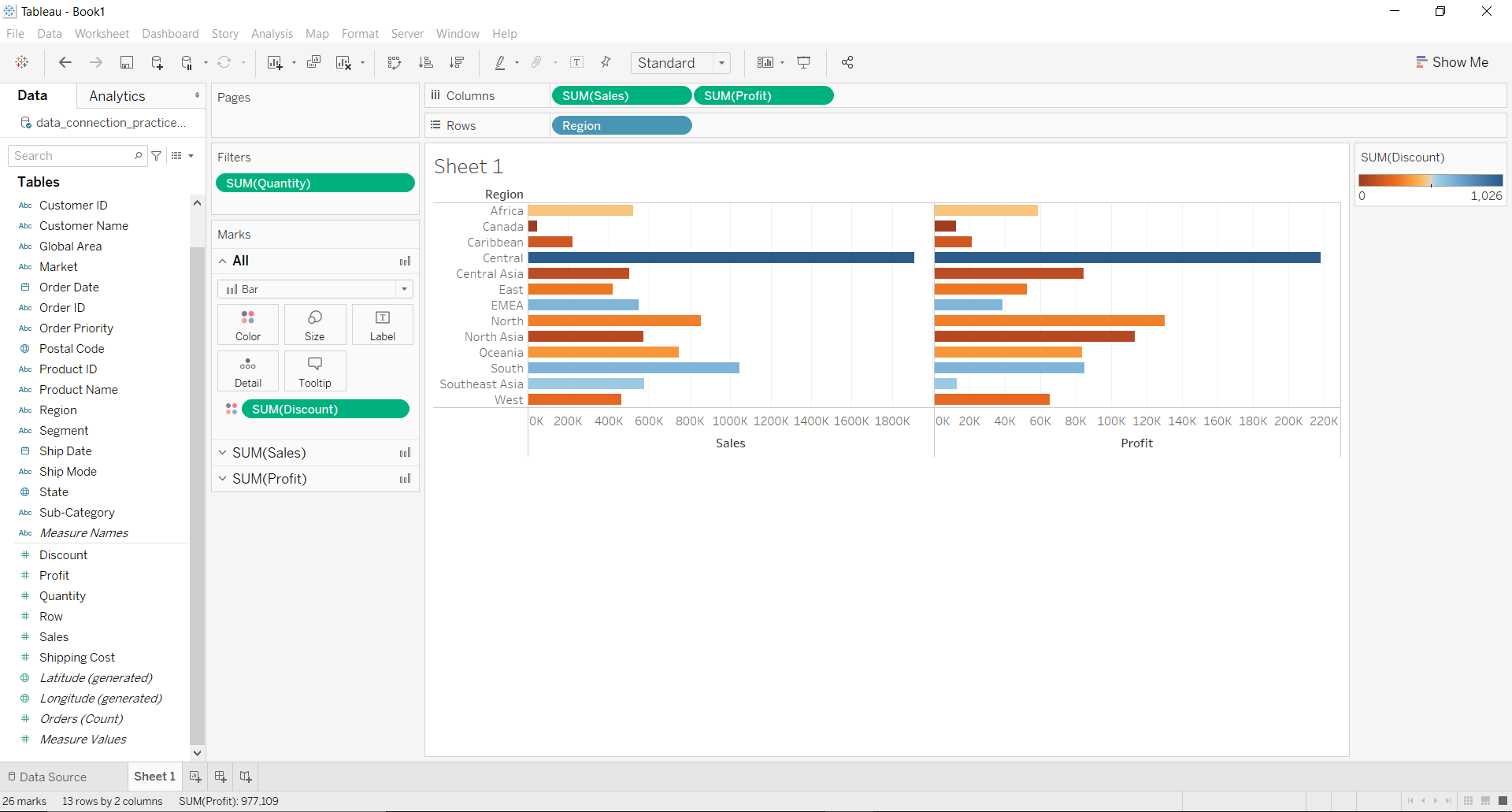My Personal Notes arrow_drop_up